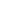#Coordinate Geometry GEO-1010 is a Course

## Coordinate Geometry GEO-1010

Self-paced

### Full course description

Course Description

Coordinate geometry uses a Cartesian coordinate system to analyze shapes such as lines or circles. In this course, you will work with a two-dimensional coordinate system.

By the end of this course, you will be able to

• Understand a two-dimensional coordinate system

• Calculate the length of a line segment

• Calculate the midpoint of a line segment

• Calculate the slope of a line

• Understand the equation of a line

• Understand the equation of a circle

Estimated completion time (hours): 1.2